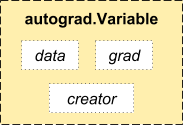Autograd 现在是 torch 自动微分的核心包 . 它是使用基于 tape 的系统来进行自动微分的.

## Variable (变量)Variable

``````import torch
print(x)  # 注意 "Variable containing" 行
``````
``````print(x.data)
``````
``````print(x.grad)
``````
``````print(x.grad_fn)  # 我们自己创建的 x
``````

``````y = x + 2
print(y)
``````

``````print(y.grad_fn)
``````

``````z = y * y * 3
out = z.mean()

print(z, out)
``````

## 梯度

``````out.backward()
``````

``````x = Variable(torch.ones(2, 2), requires_grad=True)
y = x + 2
y.backward(torch.ones(2, 2), retain_graph=True)
# retain_variables 标志将阻止内部缓冲区被释放
``````
``````z = y * y
print(z)
``````

``````gradient = torch.randn(2, 2)

# 如果我们没有指定我们想保留变量, 这将会失败